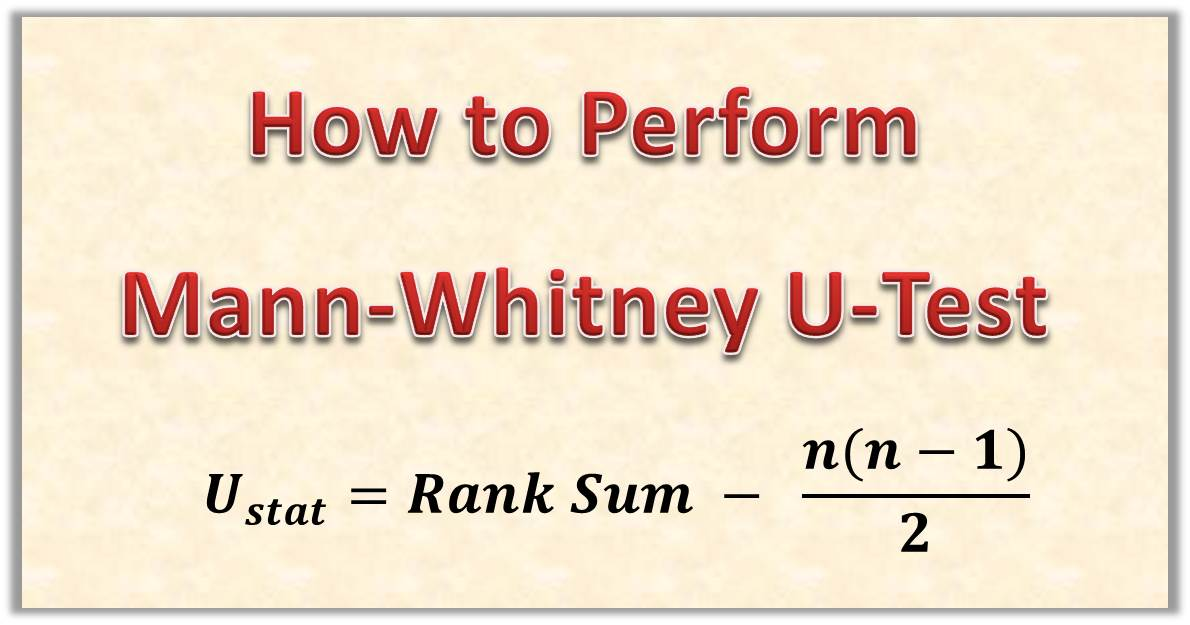# How to Perform Mann-Witney U Test(Step by Step) – Hypothesis TestingThe Mann-Witney U Test is is an interesting procedure and really very straightforward if you would follow through. I happy to know that you are making effort to learn hypothesis testing.

Feel free to leave me a comment below if you have any challenges. I would be happy to give you needed support.

Content

### 1. What is Mann-Whitney u-Test?

Mann-Whitney u-Test is a non-parametric test used to test whether two independent samples were selected from population having the same distribution. Another name for the Mann-Whitney U Test is Wilcoxon Rank Sum Test.

Note: This is not the same as Wilcoxon Signed Rank Test which is used for dependent samples.

Just as you know, the easiest way to understand as statistical test is to just perform the test yourself. So now we are going to go through an example and be sure to follow along with a pen,  notebook and a calculator. It is really easy and fun!

### 2. Exercise

A  researcher gave an aptitude test to 24 respondents, 12 were men and 12 of them were women. He recorded the scores for each of the responded and tabulated it in the table below:

 Men 80 79 92 65 83 84 95 78 81 85 73 52 Women 82 87 89 91 93 76 74 70 88 99 61 94

Use thid data provided to test the null hypothesis that the distribution of scores is the same for men as for women. Use a significance level of 0.05. (Use the Wilcoxon Rank Sum Test)

### 3. Solution Steps

We would follow the step by step procedure. We would also use excel to tabulate our data to make it easier to perform calculation.

#### Step 1: State the null and alternate hypothesis and rejection criteria

The null hypothesis states that median difference between the pairs ranks of the observations is zero (that is there is no difference in the ranks of the two pairs of observations) and the alternate hypothesis states that the median difference between the ranks of data is not zero.

These are stated below.

H0: μm = μw
H1: μm ≠ μw

Alpha = 0.05

Rejection Criteria: Reject the null hypothesis if Ustat < Ucrit

#### Step 2: Perform a ranking of all the observation

In this case, we simply subtract corresponding pairs of the data.
We would used MS Excel to help us do it faster. So, I have transferred the table excel and the added a column for the difference in the two pairs of observations.

Total number of observation is 24, so we need to rank the observations from 1 to 24. In the figure, I have colored the observation for Men as blue and for Women as red. This is so that, when I sort the data, I would know which one belongs to which group.

I sorted the data to make it easy to assign the ranks to each of the observation.

#### Step 3: Calculate the Rank Sums

We calculate the sum of the ranks for the two groups

Sum for Men (n1) = 10+9+20+3+13+14+23+8+11+15+5+1 = 132

Sum for Women (n2) = 12=16+18+19+21+7+6+4+17+24+2+22 = 168

#### Step 4: Calculate the U Statistic for the Two Groups

The formula the the U statistic is given as:

For the Men observation, we have

We also calculate the U for the Women observation, we have

The U-stat is the smaller value of the two and that would be

Ustat = 66

#### Step 5:Determine the Critical value from Table

From Mann-Withney u-test table, we check the value under column 12 and row 12
We have a critical value of U to be

Ucrit = 37

Since the calculated value of U is greater than the critical value, we accept the null hypothesis and agree that the two groups are the same.